#### You may also like### Summing Consecutive Numbers

15 = 7 + 8 and 10 = 1 + 2 + 3 + 4. Can you say which numbers can be expressed as the sum of two or more consecutive integers?A picture is made by joining five small quadrilaterals together to make a large quadrilateral. Is it possible to draw a similar picture if all the small quadrilaterals are cyclic?### Angle Trisection

It is impossible to trisect an angle using only ruler and compasses but it can be done using a carpenter's square.

# Drawing Squares

##### Age 11 to 16

Thank you to everyone who has sent in solutions to this problem:

Emma, from Wycombe High School, and Kieran, from Sacred Heart Catholic College, gave good explanations as to why all the shapes are squares. Here is Kieran's solution:

They are all squares as they all have four equal length sides and the angles are all right angles.

Emma then explained why you can start with any pair of points and go on to form a square:

You can start with any two dots because the dots are in neat rows and columns. This means that any line can be transformed into another space on the grid by translating or rotating by $90^\circ$. This means you can get four line segments at right angles of equal length.

Kara, from Highgate School, Australia, and Emma both noticed that you can make a rectangle on the dotty grid.

Theo, from Malet Lambert School, found that you can also make an isosceles trapezium: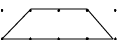Geraldine and Brendan have sent us several other shapes that they could make on a dotty grid.

Lots of other shapes can be made, with as many sides as you want. Here are some of the special quadrilaterals that we made: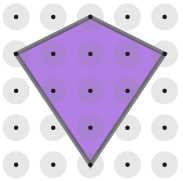”ƒ”ƒ”ƒ”ƒ”ƒ”ƒ”ƒ”ƒ”ƒ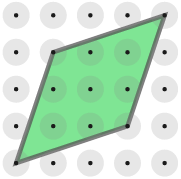”ƒ”ƒKite ”ƒ”ƒ”ƒ”ƒ”ƒ”ƒ”ƒ”ƒ”ƒ”ƒ”ƒ”ƒ”ƒ”ƒ”ƒ”ƒ”ƒRhombus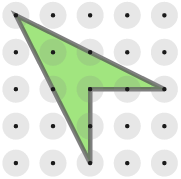”ƒ”ƒ”ƒ”ƒ”ƒ”ƒ”ƒ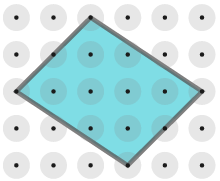Mikaeel sent us this excellent method for finding the area of these tilted squares. Great work, Mikaeel!

In order to find the area of a square on dotty paper, we first need to make the slanted sides into right-angled triangles. That way the horizontal and vertical sides will be positive integers. Let us call these sides $x$ and $y$, as below: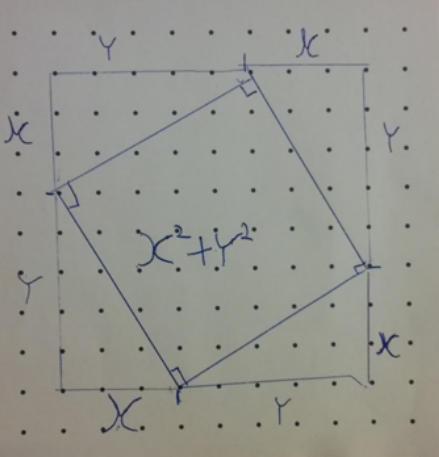We will then find that the area of the square is $x^2+y^2$. There are two ways that we can prove this; either by finding the area of the larger square and subtracting the area of the triangles or by using Pythagoras' Theorem to find the length of one of the square's sides.

Proof 1: Subtracting the triangles from the larger square

The area of the larger square is $(x+y)(x+y)=x^2 + y^2 + 2xy$

The area of one of the triangles is $\frac{1}{2}xy$. So the area of all four triangles will be $\frac{1}{2}xy\times 4 = 2xy$

So the area of the tilted square will be the area of the larger square subtract the area of all four triangles: $$x^2 + y^2 + 2xy - 2xy = x^2 + y^2$$Proof 2: Using Pythagoras

Let the length of one of the sides of the square be $l$. So the area of the square will be $l^2$, but we need to find $l$.

We know that $l$ is the hypoteneuse of these right-angled triangles. So we can use Pythagoras' Theorem to find that $x^2 + y^2 = l^2$

Therefore, the area of the rotated square is $x^2 + y^2$.

Matthew sent us this explanation of which regular polygons can be drawn. Well done, Matthew!

To find which polygons we can make, we need to consider their interior angles. for an $n$-sided polygon, its interior angles are $\frac{180(n-2)}{n}$ degrees. So this means we need to make $60^\circ$, $90^\circ$, $108^\circ$, $120^\circ$, $128.6^\circ$, $135^\circ$, etc.

However, on a square dotty grid, the only rational angles we can make are multiples of $45^\circ$. So that leaves us with $45^\circ$, $90^\circ$, $135^\circ$, ... This would suggest that we can only draw squares or octagons.

We have seen plenty of squares drawn already, so here are a couple of octagons I made.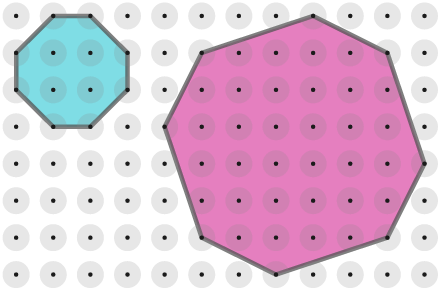These octagons both have interior angles of $135^\circ$, all of which are equal. However, when I checked the lengths of each side, they were not the same. The blue octagon has four sides of length $1$ and four sides of length $\sqrt 2$. The pink octagon has four sides of length $\sqrt 5$ and four sides of lengh $\sqrt {10}$. So I was able to make octagons with equal angles, but they did not have sides of the same length. In both cases, four of the sides were $\sqrt 2$ times longer than the other four sides, which I thought was interesting.

I then tried to make some octagons with all the sides of equal length. Here are some of the octagons I drew: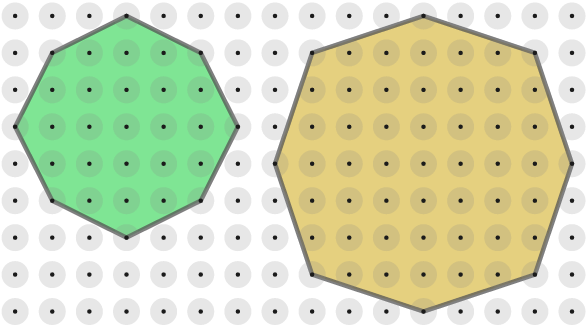Both of these octagons have sides of equal length, with the green octagon having all sides of length $\sqrt 5$ and the yellow octagon having all sides of length $\sqrt 10$. However, when I checked the size of the interior angles, they were not all the same. The green octagon had four angles of $126.87^\circ$, which were the top-, bottom-, left-, and right-most angles. The other four angles were $143.13^\circ$. All of these differed from $135^\circ$ by $8.13^\circ$. The yellow octagon had its top-. bottom-, left- and right-most angles as $143.13^\circ$ and the other angles as $126.87^\circ$. Again, these all differed from $135^\circ$ by $8.13^\circ$. So I was able to make octagons with equal sides, but they did not have angles of equal size.

Here is another octagon that I drew that is even closer to being regular: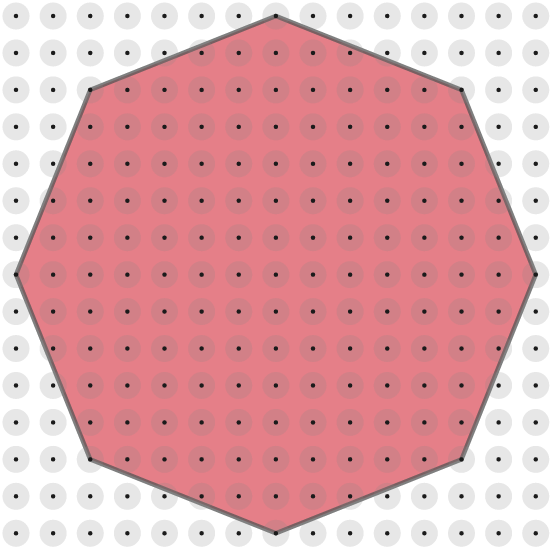This red octagon has sides of length $\sqrt {29}$, all of which are equal. Its top-, bottom, left-, and right-most angles are $136.4^\circ$ and the ohers are $133.6^\circ$. This octagon is even closer to being regular, but its angles still differ from $135^\circ$ by $1.4^\circ$.

Having gotten so close to a regular octagon, I decided to see if I could work out a way to find the line I would need to use to make an interior angle of $135^\circ$. I did this by adding some triangles like so: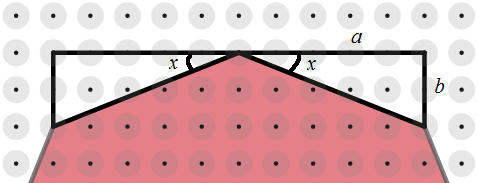To make the interior angle be $135^\circ$, I need $$180 - x - x = 135\\ 2x = 45\\ x = 22.5$$From trigonometry, I know that $\tan{x} = \frac{b}{a}$, so I need to find an $a$ and $b$ that will give me $x = 22.5^\circ$. Since we are working on a square dotty grid, I know that $a$ and $b$ must be integers, therefore $\frac{b}{a}$ must be a rational number. However, using a calculator I found that $\tan{22.5^\circ} = 0.4142... = \sqrt{2}-1$, which is an irrational number. This means that there are no integer $a$ and $b$ that would work, so it is not possible to make $x = 22.5^\circ$ on a square dotty grid.

Therefore, we cannot draw an octagon with both all sides the same and all angles the same. Therefore, the only regular polygon we can draw is a square. However, we can draw all sorts of irregular polygons.